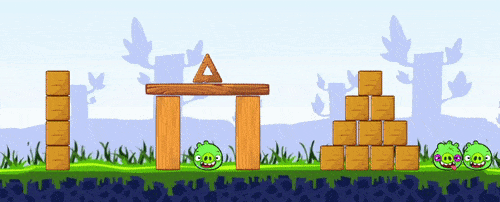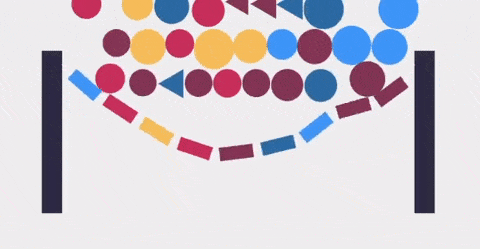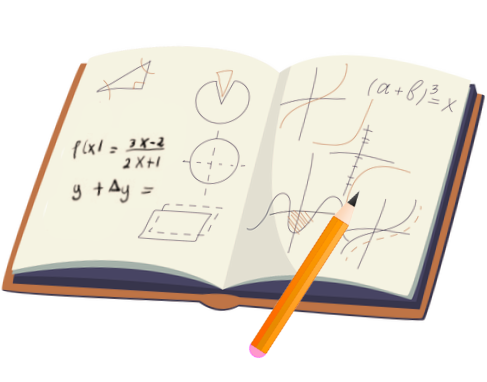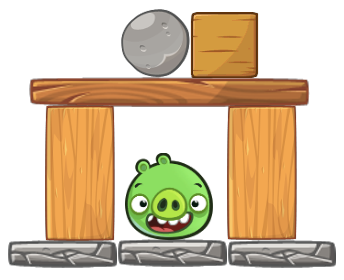## Course description

This course is a gentle introduction into the world of 2D game physics! We'll review all the beautiful math that provides the foundation for most physics engines, starting with a strong review of vectors, matrices, basic trigonometry, rigid-body collision, and touching a little bit of calculus as well.The lectures are designed to teach all concepts from first principles. In our journey, we'll review several topics from physics, like velocity, acceleration, integration, mass, forces, gravity, drag, friction, rigid body dynamics, collision detection, constraints, etc.

We'll also put theory into practice by coding a very simple 2D physics engine from scratch using the C++ programming language.

We'll start by writing a simulation of particle physics, which is a good start for us to address concepts like movement, forces, displacement, and integration.

We'll then proceed to work with rigid bodies by adding shapes to our objects, like circles, rectangles, and polygons. We'll also learn how to code the collision detection and collision resolution between these rigid bodies.We'll conclude our C++ implementation by adding constraints to our physics engine, which will help us add different types of objects to our engine, like joints and ragdolls. Ultimately, constraints will help us improve the stability of our engine, and they are a great opportunity for us to discuss some interesting ideas from calculus.

## The tools you'll need

All you really need is a simple code editor and a C++ compiler. We'll use a cross-platform library called SDL to display our graphics, and since we can find a C++ compiler for virtually any operating system, you can follow along on either Windows, macOS, or Linux!Also, make sure you have pen and paper ready for the lectures. This course will probably be a little bit different than other programming courses you took before. We will take our sweet time and make sure we understand every formula we find along the way!## Is this course for you?

This is a self-contained course with no formal prerequisites. However, you will probably get the most out of it if you already know the basics of coding (if-else, loops, functions).

We will explore some very interesting math along the way, but all you really need is to know some simple algebra. If you know how to solve the equation below for x, then you should be able to follow along and have fun like everyone else!

$$22-4x=2(3x+1)$$
view solution...

And finally, if you never programmed in C++ before... don't worry! Many successful students come from different languages like Java, Python, JavaScript, Swift, and others. We'll go over the ugly parts of C++ together.Gustavo Pezzi is a university lecturer in London, UK. He has won multiple education awards as a teacher and is also the founder of pikuma.com.

Gustavo teaches fundamentals of computer science and mathematics; his academic path includes institutions such as Pittsburg State University, City University of London, and University of Oxford.## Course content

35 hours total length 32 Chapters Last updated January 2023
• Introduction and Motivations
• How to Take this Course?
• What is Game Physics?
• A Quick Review of Vector Math
• Scalar and Vector Quantities
• Intro to the P5js Web Editor
• Coding the Vec2 Class
• Quiz: Scalars and Vectors
• Vector Magnitude
• Vector Subtraction
• Examples of Vector Addition and Subtraction
• Vector Equality
• Scaling Vectors
• Quiz: Vector Arithmetic
• Dot Product
• Is the Dot Product Commutative?
• Quiz: Dot Product
• Cross Product
• Coding the Cross Product Method
• Exercise: Perpendicular 2D Vectors
• Exercise: Vec3 Class
• Examples of Dot Product and Cross Product
• Quiz: Cross Product
• Vector Normalization
• Coding the Vec2.Normalize Method
• Scaling, Translating, and Rotating Vectors
• Review of Sine, Cosine, and Tangent
• Vector Rotation Proof (x)
• Vector Rotation Proof (y)
• Coding the Vec2 Rotate Method
• Quiz: Vector Transformations
• Concluding our JavaScript Vec2 Class
• A Review of the C++ Syntax for Vec2
• Libraries and Dependencies
• Project Folder Structure
• Initial Project Files
• Compiling our Project on Linux
• Compiling our Project on macOS
• Makefiles
• Configuring Visual Studio on Windows
• Introduction to Particle Physics
• Particle Class
• Particle Velocity
• Exercise: Operator + to Add Vectors
• Controlling our Framerate
• Framerate Independent Movement
• Variable Delta Time
• Quiz: Framerate and Delta Time
• Moving in a Constant Velocity
• Changing Particle's Velocity
• Keeping Particles Inside the Window
• Constant Acceleration
• Discrete vs. Continuous
• Integration & Simulation of Movement
• Different Notations for Differentation
• Integrals as Continuous Sum
• Different Integration Techniques
• Particle Integrate Function
• Quiz: Integration
• Applying Forces to Particles
• Kinematics vs. Kinetics
• Particles with Different Mass
• Weight Force
• Inverse of Mass
• Quiz: Forces and Mass
• Exercise: Applying Forces on Key Press
• Drag Force
• Function to Apply Drag
• Handling Mouse Clicks with SDL
• Friction Force
• Function to Apply Friction
• Examples of Forces in Games
• Quiz: Drag and Friction
• Gravitational Attraction Force
• Coding the Gravitational Attraction Force
• Quiz: Gravitational Attraction Force
• Spring Force
• Spring Force Function
• Exercise: Spring Forces
• Chain of Objects with Springs
• Soft Body with Springs
• A Discussion on Verlet Integration
• Rigid Bodies
• Different Rigid Body Shapes
• Shape Parent Class
• Circle Shape
• Angular Velocity
• Angular Acceleration
• Torque and Moment of Inertia
• Angular Motion for the Circle Shape
• Exercise: Coding Torque and Moment of Inertia
• Direction of the Angular Velocity
• Quiz: Angular Motion
• Box Vertices
• Local Space vs. World Space
• Body Update Function
• Quiz: Local Space and World Space
• Circle-Circle Collision Detection
• Collision Detection Class
• Collision Contact Information
• Coding the Circle-Circle Collision Information
• Broad Phase and Narrow Phase
• The Projection Method
• Objects with Infinite Mass
• Floating-Point Comparison
• Quiz: Circle-Circle Collision Detection
• Impulse Method and Momentum
• Impulse
• Deriving the Linear Impulse Formula
• Coding the Linear Impulse Function
• Is Linear Collision Resolution Good Enough?
• Quiz: Linear Collision Resolution
• AABB Collision
• SAT: Separating Axis Theorem
• Different SAT Approaches
• Finding the Minimum Separation with SAT
• Polygon-Polygon Collision Code
• What are Support Points?
• Finding Collision Information with SAT
• Quiz: SAT
• Linear and Angular Velocity at a Point
• Post-Collision Velocity at a Point
• Computing the Linear & Angular Velocity at a Point
• Collision Distance Vectors ra and rb
• 2D Cross Product Simplification
• Impulse Along the Normal Vector
• Exercise: Impulse Along the Tangent Vector
• Friction Along the Tangent Vector
• Discussing Collision Resolution in 3D
• Circle-Polygon Collision Detection
• Finding Polygon's Nearest Edge with Circle
• Exercise: Computing Circle-Polygon Edge Regions
• Exercise: Polygons with More than 4 Vertices
• Quiz: Circle-Polygon Collision
• Rendering Circle Texture
• Rigid Body Textures
• Creating a World Class
• Coding the World Functions
• Refactoring Function to Update Vertices
• Local Solvers vs. Global Solvers
• A Naive Iterative Solution to Positional Correction
• Quiz: Local Solvers vs. Global Solvers
• Constrained Rigid Body Physics
• Position Constraint vs. Velocity Constraint
• Example of Velocity Constraint and Bias Factor
• Force Constraint & Constrained Movement
• Force-Based vs. Impulse-Based Constraints
• Coding the Constraint Parent Class
• Quiz: Constraints
• VecN Class
• VecN Functions
• Matrices
• MatMN Class
• MatMN Functions
• Matrix Transpose
• Matrix Multiplication Function
• Exercise: Seeing Beyond the Matrix
• Generalized Velocity Constraint
• Solving Violated Velocity Constraint
• Constraint Class Inheritance
• Distance Constraint
• Joint Constraint Class
• Joint Constraint vs. Distance Constraint
• Exercise: Converting from World Space to Local Space
• Inverse Rotation Matrix
• Deriving the Distance Jacobian
• Solving the System of Equations (Ax=b)
• The Gauss-Seidel Method
• Constrained Pendulum
• Quiz: Distance Constraints
• Solving System of Constraints Iteratively
• Warm Starting
• Exercise: Ragdoll
• Quiz: System of Constraints
• Penetration Constraint
• Deriving the Penetration Jacobian
• Coding the Penetration Constraint Class
• Solving Penetration Constraints
• Linear Complementary Problem
• Penetration Warm Starting
• Quiz: Penetration Constraint
• Penetration Constraint Friction
• Clamping Friction Values
• Unstable Stack of Boxes
• Allowing for Multiple Contact Points
• Reference Edge and Incident Edge
• Finding the Incident Edge
• Clipping
• Coding the Clipping Function
• Testing Multiple Objects and Multiple Constraints
• Quiz: Stack of Boxes
• Contact Caching
• Continuous Collision Detection
• Computing the Moment of Inertia of Polygons
• Split Between Broad Phase and Narrow Phase
• Conclusion
• Moving Forward

## How is this course different?This course is not just a simple tutorial on how to use a physics engine like Box2D or Bullet. This course focus on the foundation of what makes physics engines tick, including a comprehensive review of the beautiful math that comes with it.

While you can find other resources on game physics out there, most of them are either too theoretical or overwhelmingly complex. This course is a beginner-friendly introduction to the world of game physics, providing a mix between the theory we'll see in the lectures with the practical examples we'll write using C++.

So, if you ever wanted to understand what happens under the hood of a physics engine, then get ready and buckle up! This is going to be a 35-hour journey of pure nerd fun.

73% of our students come back for another courseWe don't offer discounts on our courses. Ever.

## What students are saying

4.96
 5 star 96.0% 4 star 4.0% 3 star 0.0% 2 star 0.0% 1 star 0.0%
"Probably one of the best courses I've ever taken. Extremely informative, well taught and made. A great base from which to start learning more advanced game physics topics. A great introduction to 2d game physics programming!"
16 Nov 2023
"Absolutely amazing! Just a wonderful course from start to finish. I can't speak highly enough of the content or its delivery. If you've ever pondered what goes into a 2D physics engine, and if you've ever found yourself wishing someone could explain things in the simplest (and best) possible terms, look no further. Gustavo is a remarkable educator, and this course is worth your time and effort. I have loved every moment of it."
31 Oct 2023
Marcos Arribas
"Great course! On average, I would say that everything is good, but what I really liked, is that is very well paced (fast enough to see the improvements and keep the motivation, but still not rushing the explanations)"
25 Oct 2023Washington Irving
"Best! Just great. Gustavo is a great and charismatic teacher. I learned a lot."
30 Sep 2023

## Other similar courses

### C++ 2D Game Engine Development

30 hours
• Learn to make a simple 2D game engine using modern C++, SDL, ECS, and Lua.

### 3D Computer Graphics Programming

35 hours
• Create a 3D software renderer from scratch using the C programming language.

### Raycasting Engine Programming with C

18 hours
• Write a raycasting engine with textures and sprites using the C programming language.